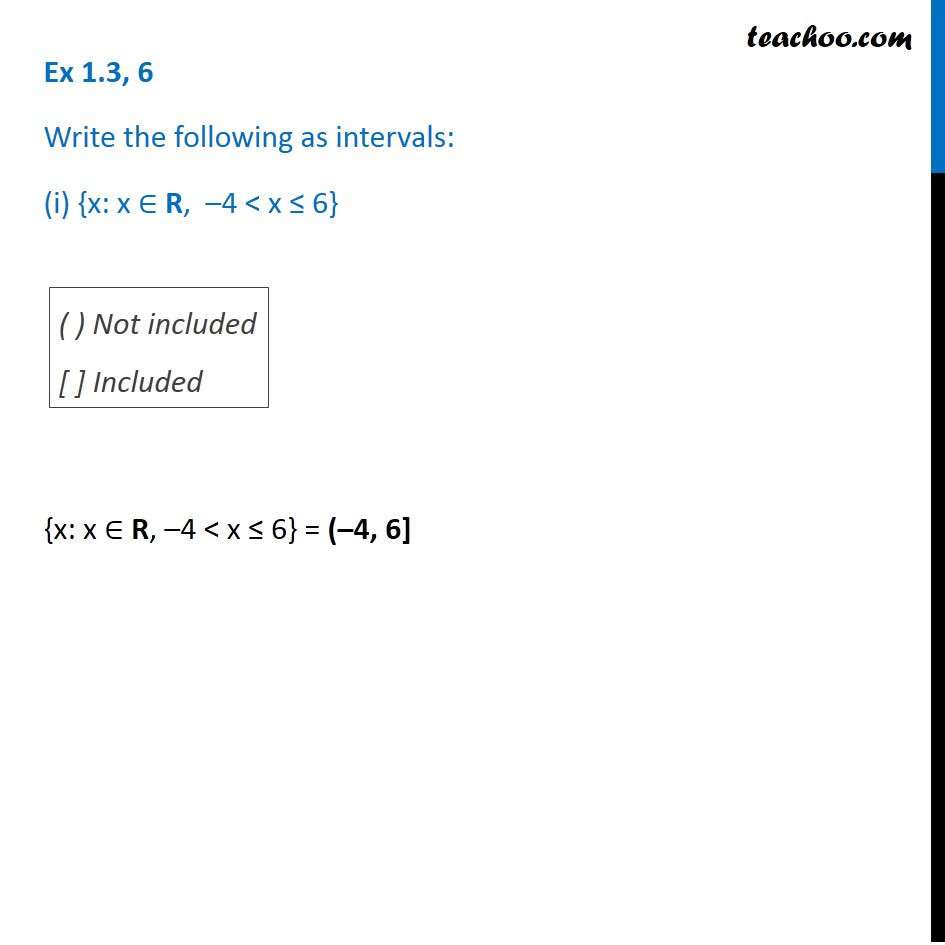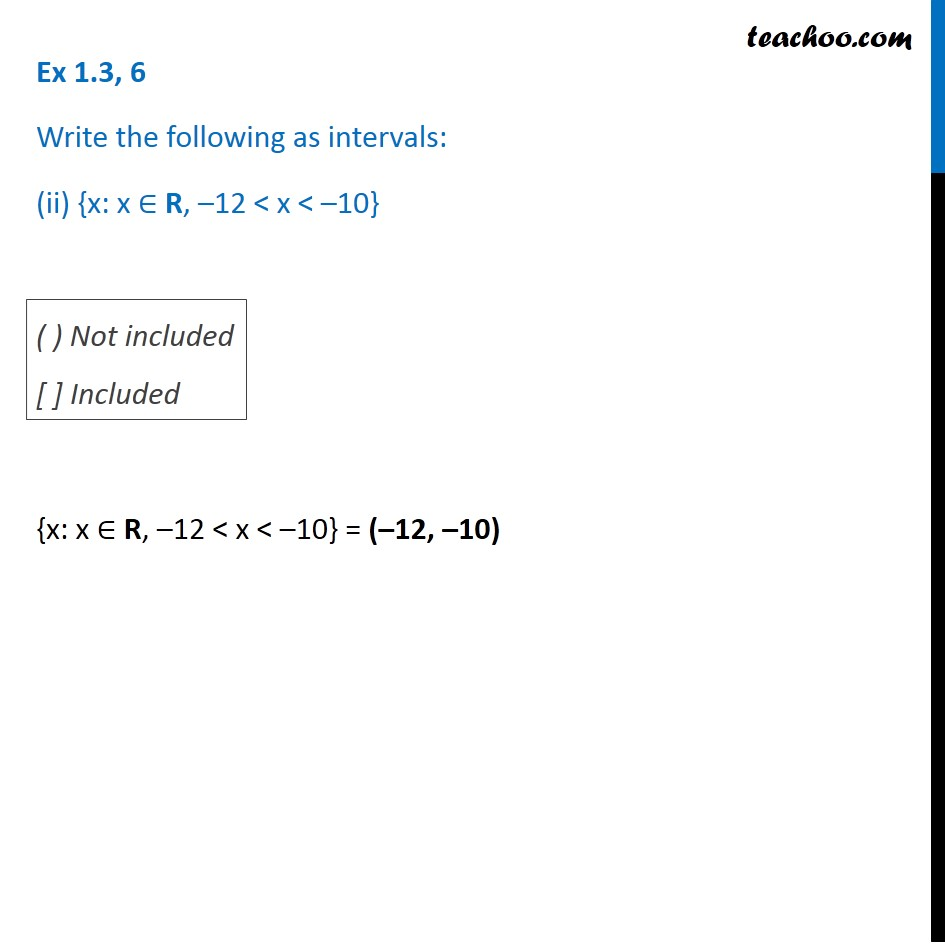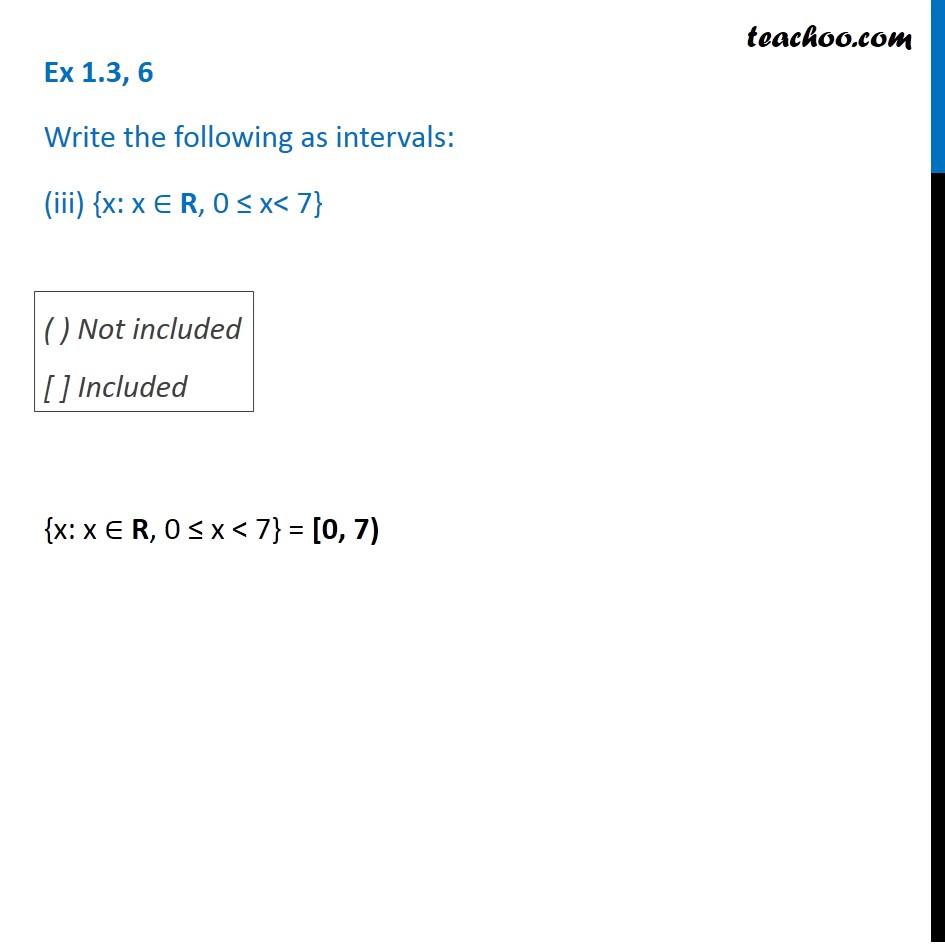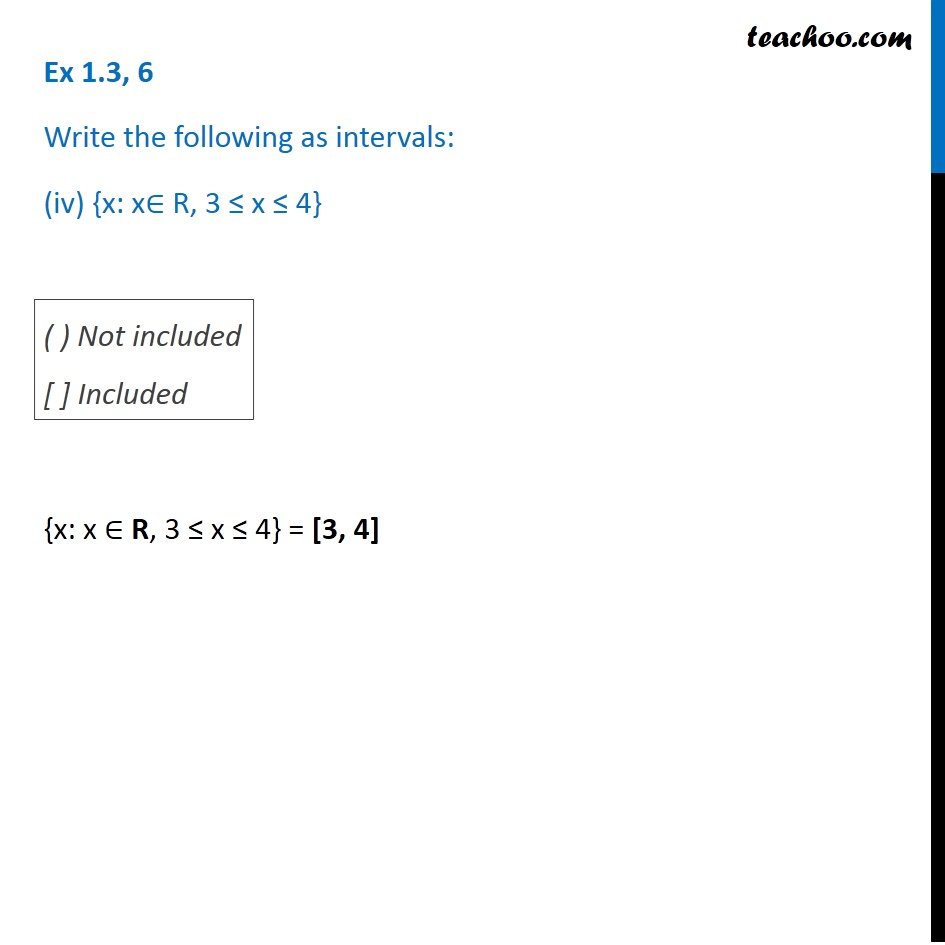1. Chapter 1 Class 11 Sets (Term 1)
2. Serial order wise
3. Ex 1.3

Transcript

Ex 1.3, 6 Write the following as intervals: (i) {x: x ∈ R, –4 < x ≤ 6} {x: x ∈ R, –4 < x ≤ 6} = (–4, 6] ( ) Not included [ ] Included Ex 1.3, 6 Write the following as intervals: (ii) {x: x ∈ R, –12 < x < –10} {x: x ∈ R, –12 < x < –10} = (–12, –10) ( ) Not included [ ] Included Ex 1.3, 6 Write the following as intervals: (iii) {x: x ∈ R, 0 ≤ x< 7} {x: x ∈ R, 0 ≤ x < 7} = [0, 7) ( ) Not included [ ] Included Ex 1.3, 6 Write the following as intervals: (iv) {x: x∈ R, 3 ≤ x ≤ 4} {x: x ∈ R, 3 ≤ x ≤ 4} = [3, 4]

Ex 1.3